# New Pattern Quantitative Aptitude Questions Based on IBPS PO Mains 2018 (Day-03)

Dear Aspirants, Quantitative Aptitude plays a crucial role in Banking and all other competitive exams. To enrich your preparation, here we have provided New Pattern Aptitude Questions that are similar to the questions asked in IBPS PO Mains 2018. Candidates those who are going to appear in upcoming bank exams like IBPS Clerk, IBPS SO, etc., can practice these questions daily and make your preparation effective.

[WpProQuiz 4532]

Directions (Q. 1 – 5): The questions below are based on the given Series-I. The series-I satisfy a certain pattern, follow the same pattern in Series-II and answer the questions given below.

1)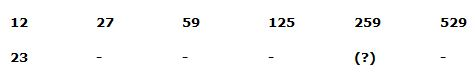a) 427

b) 463

c) 435

d) 297

e) 623

2)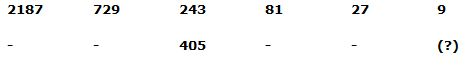a) 18

b) 15

c) 16

d) 12

e) 21

3)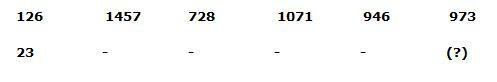a) 961

b) 689

c) 790

d) 870

e) 890

4)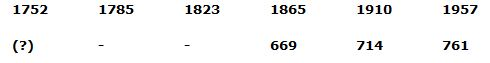a) 556

b) 456

c) 486

d) 526

e) 429

5)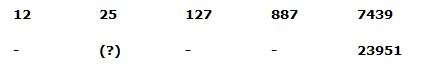a) 121

b) 119

c) 113

d) 117

e) 111

Directions (6 – 10): Study the following graph carefully and answer the given questions.

The table shows the discount rate of four different items in five different shops.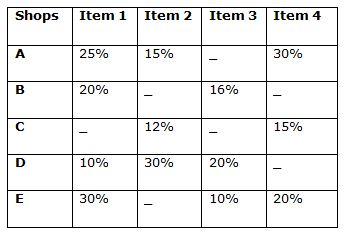Note: Selling price for each item is same in all the shops

6) Quantity I: If the ratio of the marked price of item 1 and item 4 in shop E is 15: 14, and the marked price of shop D in item 1 is Rs. 7000. Find the selling price of item 4 in shop E?

Quantity II: In item 2, if marked price in shop C is 25% more than the cost price and the profit percentage of shop C is 10% which is equal to 280. Find the cost price of shop D if marked price of item 2 in shop D is 10% more than the cost price?

a) Quantity I > Quantity II

b) Quantity I ≥ Quantity II

c) Quantity I < Quantity II

d) Quantity I ≤ Quantity II

e) Quantity I = Quantity II (or) Relationship cannot be determined

7) Quantity I: Item 3, cost price of all the shops is Rs. 4800 and the marked price is 40%, 50% and 25% more than the cost price in shop B, D and E respectively. Find the total selling price of shop B, D and E together

Quantity II: If the selling price of item 4 of all the shops is Rs. 9520, find the total marked price of shop A, C and E?

a) Quantity I > Quantity II

b) Quantity I ≥ Quantity II

c) Quantity I < Quantity II

d) Quantity I ≤ Quantity II

e) Quantity I = Quantity II (or) Relationship cannot be determined

8) Quantity I: In shop E, Cost price of item 1 is Rs. 800 more than the cost price of item 4 and the marked price of item 1 and 4 is 60 % and 40 % more than the cost price respectively. Find the selling price of item 4 if the selling price is same for both the items?

Quantity II: If the marked price of item 3 in shop B is 20% more than the cost price, which is 6000, find the marked price of item 3 in shop D?

a) Quantity I > Quantity II

b) Quantity I ≥ Quantity II

c) Quantity I < Quantity II

d) Quantity I ≤ Quantity II

e) Quantity I = Quantity II (or) Relationship cannot be determined

9) In shop D, if the ratio of marked price in item 1, item 2 and item 3 is 56: 72: 63 and the marked price of item 1 in shop B is Rs.6300.

Quantity I: Find the marked price of item 2 in shop A

Quantity II: Find the marked price of item 3 in shop E

a) Quantity I > Quantity II

b) Quantity I ≥ Quantity II

c) Quantity I < Quantity II

d) Quantity I ≤ Quantity II

e) Quantity I = Quantity II (or) Relationship cannot be determined

10) Quantity I: Find the marked price of item 4 in shop A. If the marked price of item 4 in shop E is Rs. 6300

Quantity II: Find the marked price of item 2 in shop C. If the marked price of item 2 in shop D is Rs. 4400

a) Quantity I > Quantity II

b) Quantity I ≥ Quantity II

c) Quantity I < Quantity II

d) Quantity I ≤ Quantity II

e) Quantity I = Quantity II (or) Relationship cannot be determined

Directions (1-5):

Series I pattern:

12*2 + 3 = 27

27*2 + 5 = 59

59*2 + 7 = 125

125*2 + 9 = 259

259*2 + 11 = 529

Series II pattern:

23*2 + 3 = 49

49*2 + 5 = 103

103*2 + 7 = 213

213*2 + 9 = 435

435*2 + 11 = 881

Series I pattern:

2187 ÷ 3 = 729

729 ÷ 3 = 243

243 ÷ 3 = 81

81 ÷ 3 = 27

27 ÷ 3 = 9

Series II pattern:

3645 ÷ 3 = 1215

1215 ÷ 3 = 405

405 ÷ 3 = 135

135 ÷ 3 = 45

45 ÷ 3 = 15

Series I pattern:

126 + 113 = 1457

1457 – 93 = 728

728 + 73 = 1071

1071 – 53 = 946

946 + 33 = 973

Series II pattern:

23 + 113 = 1354

1354 – 93 = 625

625 + 73 = 968

968 – 53 = 843

843 + 33 = 870

Series I pattern: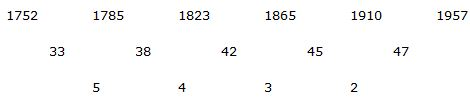Series II pattern: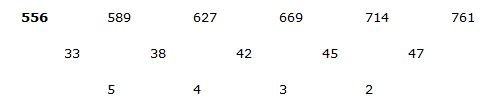Series I pattern:

12*2 + 13 = 25

25*4 + 33 = 127

127*6 + 53 = 887

887*8 + 73 = 7439

Series II pattern:

55*2 + 13 = 111

111*4 + 33 = 471

471*6 + 53 = 2951

2951*8 + 73 = 23951

Directions (6-10):

Quantity I: If the ratio of the marked price of item 1 and item 4 in shop E is 15: 14, and the marked price of shop D in item 1 is Rs. 7000. Find the selling price of item 4 in shop E?

Selling price of item 1 in shop D = 7000 * [(100 – 10)/100]

= > 7000*(90/100) = Rs. 6300

Marked price of item 1 in shop E = [6300/(100-30)]*100

= > 6300/70 * 100 = 9000

Marked price of item 4 in shop E = 9000 * (14/15) = 8400

Selling price of item 4 in shop E = 8400 * [(100-20)/100]

= > 8400 * 80/100 = Rs. 6720

Quantity II: In item 2, if marked price in shop C is 25% more than the cost price and the profit percentage of shop C is 10% which is equal to 280. Find the cost price of shop D if marked price of item 2 in shop D is 10% more than the cost price?

Profit of item 2 in shop C = Rs. 280 = 10% of cost price of item 2 in shop C

Cost price of item 2 in shop C = 280*(100/10) = 2800

Marked price of item 2 in shop C = 2800 * [(100 + 25)/100]

= > 2800 * 125/100 = 3500

Selling price of item 2 in shop C = 3500 * (100 – 12)/100

= > 3500 * (88/100) = Rs. 3080

Marked price of item 2 in shop D = 3080 * (100/(100-30))

= > (3080/70)*100= 4400

Cost price of item 2 in shop D = (4400/110)*100 = Rs. 4000

Quantity I > Quantity II

Quantity I: Item 3, cost price of all the shops is Rs. 4800 and the marked price is 40%, 50% and 25% more than the cost price in shop B, D and E respectively. Find the total selling price of shop B, D and E together

Marked price of item 3 in shop B = 4800 * (140/100) = 6720

Marked price of item 3 in shop D =4800*(150/100) = 7200

Marked price of item 3 in shop E =4800* (125/100) = 6000

Selling price of item 3 in shop B = 6720 * [(100-16)/100] = 5644.8

Selling price of item 3 in shop D = 7200 * [(100-20)/100] =5760

Selling price of item 3 in shop E = 6000 * [(100-10)/100] = 5400

Required total = (5644.8 + 5760 + 5400) = Rs. 16804.4

Quantity II: If the selling price of item 4 is Rs.9520, find the total marked price of shop A, C and E

Marked price of item 4 in shop A = 9520 * (100/70) = 13600

Marked price of item 4 in shop C = 9520 *(100/85) = 11200

Marked price of item 4 in shop E = 9520 * (100/80) = 11900

Required total = (13600+11200+11900) = Rs. 36700

Quantity I < Quantity II

Quantity I: In shop E, Cost price of item 1 is 800 more than the cost price of item 4 and the marked price of item 1 and 4 is 60 % and 80 % more than the cost price respectively. Find the selling price of item 4 if the selling price is same for both the items?

Cost price of item 4 in shop E = x

Cost price of item 1 in shop E = x+800

Marked price of item 1 in shop E = (x+800) * [(100+60)/100]

=> (x + 800)*(160/100) = (x + 800)*(8/5) = (8x + 6400)/5

Marked price of item 4 in shop E = x * (100 + 80)/100

= > (x)*(180/100)

Selling price of item 1 in shop E = (2x+1600) * [(100-30)/100]

= > [(8x + 6400)/5] * 70/100

= > [(8x + 6400)/5]*(7/10)

Selling price of item 4 in shop E = (x) * (180/100) * [(100-20)/100]

= > (x) * (180/100) * (4/5)

= > 36x/25

According to the question,

Selling price of both the items is same

[(8x + 6400)/5]*(7/10) = 36x/25

56x + (6400*7) = 72x

16x = (6400*7)

X = 2800

Selling price of item 4 in shop E = 36x/25 = (36/25)*2800 = Rs. 4032

Quantity II: If the marked price of item 3 in shop B is 20% more than the cost price, which is 6000, find the marked price of item 3 in shop D

Cost price of item 3 in shop B = 6000

Marked price of item 3 in shop B = 6000 * 120/100 = 7200

Selling price of item 3 in shop B = 7200 * [(100-16)/100]

= > 7200*(84/100) = 6048

Marked price of item 3 in shop D = 6048 * (100/80) = Rs. 7560

Quantity I < Quantity II

In shop D, if the ratio of marked price in item 1, item 2 and item 3 is 56: 72: 63 and the marked price of item 1 in shop B is Rs. 6300.

Selling price of item 1 in shop B = 6300 * (100-20)/100

=> 6300 * 80/100 = 5040

Marked price of item 1 in shop D = 5040 *(100/100-10)

=> 5040 * 100/90 = 5600

Marked price of item 2 in shop D = 5040 * (100/100-30)

= > 5040 *(100/70) = 7200

Marked price of item 3 in shop D = 5040 * (100/100-20)

= > 5040 * (100/80) = 6300

Quantity I: Find the marked price of item 2 in shop A

Selling price of item 2 in shop D = 7200 * [(100 – 30)/100]

=> 7200 * (70/100) = 5040

Marked price of item 2 in shop A = 5040 * (100/85) = Rs. 5929.4

Quantity II: Find the marked price of item 3 in shop E

Selling price of item 3 in shop D = 6300 * (100-20)/100

= > 6300 * (80/100) = 5040

Marked price of item 3 in shop E = 5040 * (100/90) = Rs. 5600

Quantity I > Quantity II

Quantity I: Find the marked price of item 4 in shop A. If the marked price of item 4 in shop E is Rs. 6300

Selling price of item 4 in shop E = 6300 * (100 – 20)/100

= > 6300 * 80/100 = 5040

Marked price of item 4 in shop A = 5040 * (100/70) = Rs. 7200

Quantity II: Find the marked price of item 2 in shop C. If the marked price of item 2 in shop D is Rs.4400

Selling price of item 2 in shop D = 4400 * (100-30)/100

=> 4400 * 70/100 = 3080

Marked price of item 2 in shop C = 3080 * (100/88) = Rs. 3500

Quantity I > Quantity II